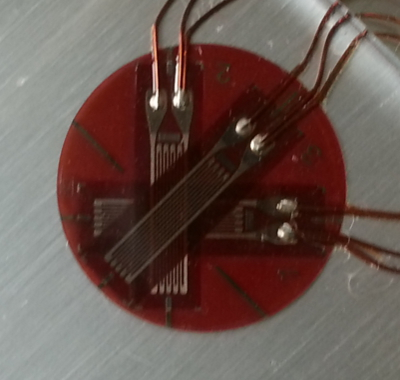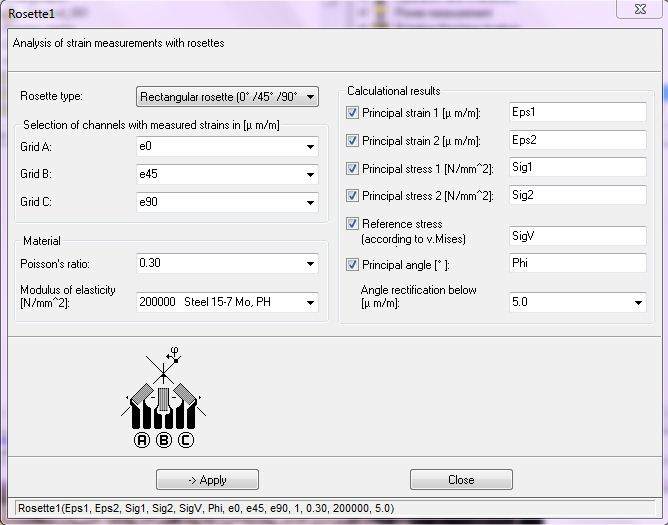Plane strain measurement where stress direction is unknown can be readily measured with Strain Rosettes. Rosettes are orthogonal strain gauges arranged at 0°, 45° and 90° at one point.

Due to the orientation of gauges and unknown stress direction, it is more practical to use quarter bridge circuit for rosette measurements.

In imc ONLINE FAMOS, there are built-in functions for rosette calculation. Two functions are provided, Rosette1 and Rosette2 with user-friendly assistants.Alternatively, if required, rosette calculations can be easily scripted in imc FAMOS Software.

Here is imc FAMOS Script for calculation of Principal Stresses (feel free to copy and paste at your disposal):-

C1 = 2 * e45 - e0 - e90           ; strain values 45, 0, 90 degree etc
C2 = e0 - e90
Theta = 0.5 * atan2( C1, C2 )     ; Principal Angle

Beta1 = 1e-6 * 0.5 * EM /(1-nu)   ; EM = Modulus of Elasticity
Beta2 = 1e-6 * EM / (1 + nu)      ; nu = Poission ratio

S1= Beta1 * (e0 + e90) + Beta2 * sqrt( (( e0-e45)^2 + (e45-e90)^2)/2)

S2= Beta1 * (e0 + e90) - Beta2 * sqrt( (( e0-e45)^2 + (e45-e90)^2)/2)

### Legal Information

Except otherwise notified, the content of this website, including the text, documents, graphics and images are copyrighted by Invicom Test & Measurement Sdn Bhd or its partner companies. All copyrights and other intellectual property rights are reserved.